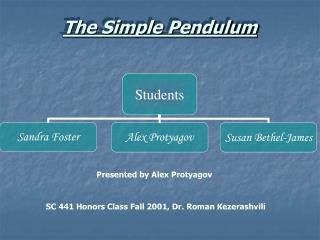DownloadDownload PresentationThe Simple Pendulum

# The Simple Pendulum

Télécharger la présentation## The Simple Pendulum

- - - - - - - - - - - - - - - - - - - - - - - - - - - E N D - - - - - - - - - - - - - - - - - - - - - - - - - - -
##### Presentation Transcript

1. The Simple Pendulum Presented by Alex Protyagov SC 441 Honors Class Fall 2001, Dr. Roman Kezerashvili

2. Objectives of the experiment: • To investigate the properties of a pendulum • To make an experimental determination of the acceleration due to gravity.

3. Pivot point Θ L Bob m m m A B The theory • The motion of a simple pendulum is periodic motion. The mass starts at point A, moves to point B where this mass starts moving in opposite direction, back to point A. For the simple pendulum all the mass is considered to be concentrated at the center. The vibration of the simple pendulum is not (in general) a SHM, but approaches SHM when the amplitude of the vibration is small compared to the length of the pendulum. • Here length, L of the pendulum is distance from a pivot point to the center of a bob. Θ is the angular displacement through which the pendulum swings and m is mass of the pendulum bob. The time, which is required for one complete vibration of oscillation, from position A to position B & back to Ais period of the pendulum, T.

4. θ L T x m θ θ m S • There are two forces acting on the bob: weight W=mg & tension T in the string. When the string makes an angle θ with the vertical, the weight has the components mgcosθ, along the string, and mgsinθ perpendicular to the string. The bob oscillates because there is restoring force. This force is resultant of the gravity and the torsion in the string. The restoring force F is the tangential component of the net force: F=-mg·sinθ mgcosθ W=mg 1 F=-mgsinθ From math we know than the sinθ≈θ when the angle θ is small. Therefore 2 F=-mgθ From figure & the definition of an angle in radians (θ= arc length/radius) 3 Here s is path length followed by the bob. This path s is curved, but when θ is pretty small, the arc length s is approximately equal to a chord x. Therefore 4 5

5. If Newton’s second law is applied to the motion of the pendulum bob, we get: 6 7 8 9 Acceleration is also this where Equation 8 shows that the small oscillations of a simple pendulum can be considered as simple harmonic motion, The constant ω represents the angular frequency of simple harmonic motion and thus the period of oscillation of the simple pendulum is 10 11 Hence The period is independent of the bob’s mass. Really pendulum will have some depends on the amplitude and therefore it may be shown as 12 This series is going smaller as in continues.

6. The pendulum can be used as a very simple device to measure the acceleration due to gravity at a particular location. We may use equation 1 to find g. Solving for g we obtain: 10

7. The period dependence on length Length L, m Period T, s % error for T Square of period T2, s2 g from equation 13, m/s2 Mean value for g from equation 13, m/s2 g from equation 14, m/s2 Standard value of g, m/s2 % error for g from equation13 % error for g from equation14 Experimental period T, s Theoretical period T from eq 11,s 0.30 1.095 1.099 0.39 1.20 9.88 9.811 9.834 9.807 0.04 0.28 0.40 1.275 1.269 0.44 1.63 9.71 0.60 1.557 1.555 0.15 2.42 9.77 0.80 1.786 1.795 0.51 3.19 9.90 1.00 2.008 2.007 0.05 4.03 9.79

8. 12

9. Summary • At this experiment we found out that the period of the simple pendulum is independent from the mass of the bob. At the first part of the experiment we find acceleration due to gravity by different ways, we compare our results with standard value, found the percent error and those numbers were pretty small. We plotted two graphs; there are “The period vs. length” &”The length vs. square of period”. The first graph looks like square root, it is understandable, it is following from equation 11th. The second graph looks like straight line, which is following from equation 11th too. As we had seen at the second part of the experiment, for small angles, smaller 10 degree, we may neglect of sin and we may use formula 11th to find the period. But for angles, which are greater formula 11 is not as good as for small angles. It is follow from second table. When we increase the angle the percent error is increasing too. Therefore for big angles is better to use formula 12.

10. Thank you for your attention